# Problem 58229. Easy Sequences 112: Almost Golden Integer Rectangles 1

A golden rectangle is a rectangle whose side lengths are in the golden ratio,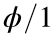, where: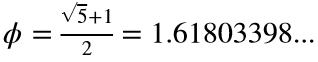.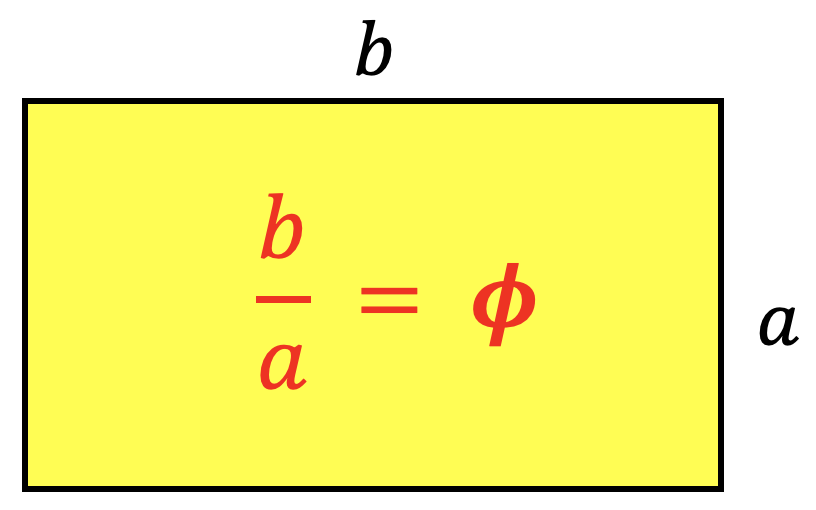The ancient Greeks consider the golden rectangle as the most aesthetically pleasing rectangular shape. Unfortunately for the ancients this ratio cannot be achieved if the sides are rational numbers let alone integers.
In this problem we are asked to find the perimeter of the rectangle with integer sides with area within the given area interval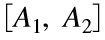, and in which the side ratio is as close as possible to the golden ratio, that is the absolute value,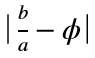, is minimized.
For example, if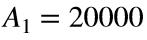and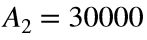, the correct dimensions should be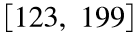, therefore the perimeter should be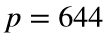:
>> A1 = 20000; A2 = 30000; phi = (sqrt(5)+1)/2; a = Inf;
>> for i = 1:A2
for j = ceil(A1/i):floor(A2/i)
r = max(j/i,i/j);
if abs(r-phi) < a
p = 2 * (i + j);
a = abs(r-phi);
end
end
end
>> p
p =
644

### Solution Stats

100.0% Correct | 0.0% Incorrect
Last Solution submitted on Sep 07, 2023

### Community Treasure Hunt

Find the treasures in MATLAB Central and discover how the community can help you!

Start Hunting!